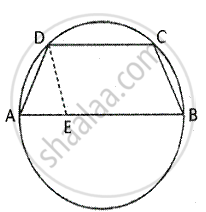Share

# If Two Non-parallel Sides of a Trapezium Are Equal, It is Cyclic. Prove It. Or an Isosceles Trapezium is Always Cyclic. Prove It. - Mathematics

Course

#### Question

If two non-parallel sides of a trapezium are equal, it is cyclic. Prove it. Or An isosceles trapezium is always cyclic. Prove it.

#### SolutionGiven – ABCD is a trapezium in which AB ∥ CD and AD = BC To prove – ABCD is cyclic

Construction – draw DE ∥ BC
Proof –
DCBE is a parallelogram         [by construction

∠DEB = ∠DCB                 [Opposite angles of parallelogram]

Also, ∠DEB = ∠EDA + ∠DAE        [Exterior angle property]

In ∠ADE, ∠DAE = ∠DAE ……. (1)   [since AD = BC = DE Or AD = DE]

Also, ∠DEB + ∠EDA =180°       ………. (2)

From (1) and (2),
∠DEB + ∠DAE =180°
⇒ ∠DCB + ∠DAE = 180°

⇒ ∠C + ∠A = 180°
Hence ABCD is cyclic trapezium

Is there an error in this question or solution?